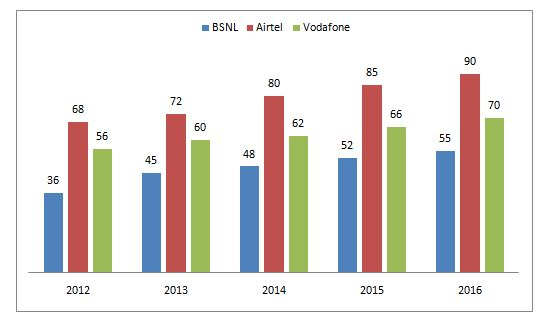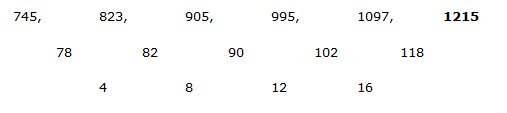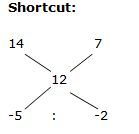# “20-20” Quantitative Aptitude | Crack IBPS RRB Assistant Prelims 2018 Day-151

Dear Readers, Find the aptitude test questions to crack latest bank exams. We regularly provide 20 aptitude test questions daily for students. Aspirants practice these questions on a regular basis to improve your score in aptitude section. Aspirants preparing for the exams can make use of this “20-20” Quantitative Aptitude Questions. Here we have started New Series of Practice Materials specially for IBPS RRB Assistant Prelims 2018. Aspirants those who are preparing for the exams can use this “20-20” Quantitative Aptitude Questions.

“20-20” Aptitude Questions | Crack IBPS RRB Assistant Prelims 2018 Day-151

Click “Start Quiz” to attend these Questions and view Solutions

Directions (1 – 5): What value should come in the place of question mark (?) in the following questions?

1) (39/153) of 15.3 % of (47/3) of ? = 1833

a) 2250

b) 1700

c) 2600

d) 3000

e) None of these

2) 1745 ÷ 34.9 + 102 × 98 – 272 =?

a) 9317

b) 8124

c) 7259

d) 9850

e) None of these

3) 175616 + (91125) % of 350 –  (262144) % of  250 = ?

a) 42.5

b) 64.5

c) 53.5

d) 60.5

e) None of these

4) 7 ¼ % of 7200 + 31 2/3 % of 5400 =? – 52 % of 700

a) 3124

b) 2596

c) 2038

d) 3780

e) None of these

5) √2809 ÷ 8 × 122 + 46 =? + 12375 ÷ 15

a) 250

b) 325

c) 175

d) 430

e) None of these

Directions (6 – 10): What value should come in the place of question mark (?) in the following number series?

6) 772, 1397, 497, 1722, 122, ?

a) 2255

b) 2364

c) 2276

d) 2381

e) 2147

7) 156, 149, 135, 114, 86, ?

a) 70

b) 51

c) 63

d) 45

e) 57

8) 9, 13, 35, 121, 509, ?

a) 2637

b) 2459

c) 2755

d) 2581

e) 2523

9) 745, 823, 905, 995, 1097, ?

a) 1215

b) 1372

c) 1348

d) 1483

e) 1289

10) 657, 656, 327, 108, ?

a) 51

b) 38

c) 26

d) 43

e) 29

11) A train crosses a platform of length 200 m in 10 sec and the same train crosses a pole in 5 sec. Find the length of the train?

a) 200 m

b) 150 m

c) 175 m

d) 250 m

e) None of these

12) Ravi has 20 % savings with him from his monthly salary. If the expenditure of clothing is 20 % of overall expenditure and his expenditure except clothing is Rs. 28000. Find his savings?

a) Rs. 5250

b) Rs. 6500

c) Rs. 7750

d) Rs. 8750

e) None of these

13) 3 years ago, Ganesh and Magathi’s age is in the ratio of 3: 4. Sum of their present ages are 7 years more than Mano’s present age. Find the present age of Mano, if Magathi’s age after 4 years is 35 years?

a) 40 years

b) 48 years

c) 28 years

d) 42 years

e) None of these

14) The Average marks obtained by 63 students in an examination is 12. If the average marks of passed students are 14 and that of failed students are 7, then find the number of students who passed the examination?

a) 38

b) 42

c) 45

d) 34

e) None of these

15) Rajesh, Meena and Chandru started a business. Rajesh invests 3 times as much as Meena and Meena invests 4/5th of Chandru’s investment. Find the share of Rajesh, if the profit at the end of the year is Rs. 136500?

a) Rs. 78000

b) Rs. 74000

c) Rs. 66000

d) Rs. 62000

e) None of these

Directions (16 – 20) Study the following information carefully and answer the given questions:

Following bar graph shows the total number of peoples (In lakhs) using three different types of mobile services over the years in a certain state.16) Find the average number of peoples using Airtel services all the given years together?

a) 83

b) 66

c) 79

d) 72

e) None of these

17) Total number of peoples using all three given services together in the year 2012 is approximately what percentage of total number of peoples using all three given services together in the year 2014?

a) 100 %

b) 85 %

c) 70 %

d) 115 %

e) 130 %

18) Find the difference between the total number of peoples using BSNL services in all the given years together to that of total number of peoples using all three given services together in the year 2013 and 2015 together?

a) 123 lakhs

b) 136 lakhs

c) 117 lakhs

d) 144 lakhs

e) None of these

19) Find the ratio between the total number of peoples using Vodafone in the year 2012, 2014 and 2016 together to that of total number of people using all three given services together in the year 2016?

a) 188: 215

b) 124: 173

c) 138: 197

d) 225: 289

e) None of these

20) Total number of people using Vodafone in the year 2013 and 2016 together is approximately what percentage of total number of people using Airtel in the year 2014?

a) 144 %

b) 152 %

c) 163 %

d) 130 %

e) 118 %

Direction (1-5)

(39/153) * (15.3/100) * (47/3) * x = 1833

X = 1833*(10*100)/(13*47)

X = 3000

(17450/349) + (102*98) – 729 = x

X = 50 + 9996 – 729

X = 9317

56 + (45/100)*350 – (64/100)*250 = x

X = 56 + 157.5 – 160

X = 53.5

(29/400)*7200 + (95/300)*5400 = x – (52/100)*700

522 + 1710 + 364 = x

X = 2596

(53/8)*12*12 + 46 = x + (12375/15)

954 + 46 = x + 825

X = 1000 – 825

X = 175

Direction (6-10)

The pattern is, +252, -302, +352, -402, +452.

The pattern is, -(1*7), -(2*7), -(3*7), -(4*7), -(5*7),…

The pattern is, *1+22, *2+32, *3+ 42, *4+ 52, *5+ 62,..The difference of difference is, 4, 8, 12, 16,…

The pattern is,

(657-1)/1 = 656, (656 – 2)/2 = 327, (327-3)/3 = 108, (108-4)/4 = 26

According to the question,

(T.L + 200)/10 = T.L/5

T.L + 200 = 2T.L

200 = T.L

The length of the train = 200 m

Savings = 20 %

Expenditure = 80 %

According to the question,

= > (80/100)*expenditure = 28000

Expenditure = Rs. 35000

(80/100)*monthly salary = 35000

Monthly salary = Rs. 43750

Savings = 20 % of monthly salary = (20/100)*43750

= > Rs. 8750

3 years ago, the ratio of ages of Ganesh and Magathi = 3 : 4 (3x, 4x)

Magathi’s age after 4 years = 35 years

Magathi’s present age = 31 years

4’s = (31-3) = 28

1’s = 7

Ganesh’s present age = 3x + 3 = 24 years

According to the question,

31 + 24 = 7 + Mano’s present age

55 – 7 = Mano’s present age

Mano’s present age = 48 years

Let the number of passed students be x,

63*12 = 14x + (63 – x)*7

756 = 14x + 441 – 7x

756 – 441 = 7x

7x = 315

Total number of passed students (x) = 45= > 5 : 2

7’s = 63

1’s = 9

Total number of passed students = 5’s = 45

The ratio of investment of Rajesh and Meena = 3: 1

The ratio of investment of Meena and Chandru = 4: 5

The ratio of investment of Rajesh, Meena and Chandru = 12: 4: 5

21’s = 136500

1’s = 6500

The share of Rajesh = 12’s = 6500*12 = Rs. 78000

Direction (16-20)

The total number of peoples using Airtel services all the given years together

= > 68 + 72 + 80 + 85 + 90

= > 395 lakhs

Required average = 395/5 = 79

Total number of peoples using all three given services together in the year 2012

= > 36 + 68 + 56 = 160 lakhs

Total number of peoples using all three given services together in the year 2014

= > 48 + 80 + 62 = 190 lakhs

Required % = (160/190)*100 = 84 % = 85 %

The total number of peoples using BSNL services in all the given years together

= > 36 + 45 + 48 + 52 + 55 = 236 lakhs

The total number of peoples using all three given services together in the year 2013 and 2015 together

= > 45 + 72 + 60 + 52 + 85 + 66 = 380 lakhs

Required difference = 380 – 236 = 144 lakhs

The total number of peoples using Vodafone in the year 2012, 2014 and 2016 together

= > 56 + 62 + 70 = 188

The total number of people using all three given services together in the year 2016

= > 55 + 90 + 70 = 215

Required ratio = 188: 215

Total number of people using Vodafone in the year 2013 and 2016 together

= > 60 + 70 = 130 lakhs

Total number of people using Airtel in the year 2014 = 80 lakhs

Required % = (130/80)*100 = 163 %

Daily Practice Test Schedule | Good Luck

 Topic Daily Publishing Time Daily News Papers & Editorials 8.00 AM Current Affairs Quiz 9.00 AM Quantitative Aptitude “20-20” 11.00 AM Vocabulary (Based on The Hindu) 12.00 PM General Awareness “20-20” 1.00 PM English Language “20-20” 2.00 PM Reasoning Puzzles & Seating 4.00 PM Daily Current Affairs Updates 5.00 PM Data Interpretation / Application Sums (Topic Wise) 6.00 PM Reasoning Ability “20-20” 7.00 PM English Language (New Pattern Questions) 8.00 PM

IBPSGuide Recommends Affairs Cloud Current affairs PDF